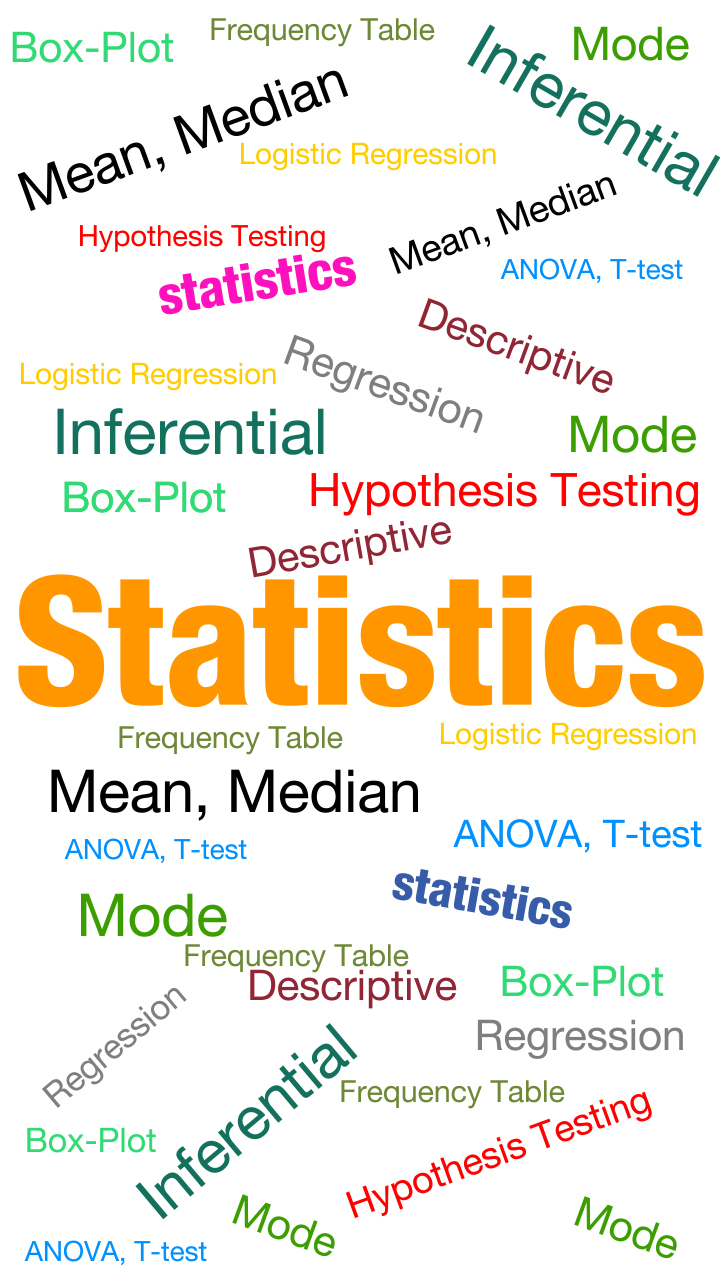Statistics is the study of the collection, analysis, interpretation, presentation, and organisation of data. Being in the field of Machine Learning and Data Science, it is paramount for a practitioner to be well versed with various statistical concepts. This guide will serve as an exhaustive resource to acquaint yourself with the various aspects of Statistics used in Data Science Industry.

Statistics learning can be categorized into three categories

• Descriptive Statistics
• Inferential Statistics
• Predictive Modeling

### Step 1: Learn Descriptive  StatisticsUdacity course on descriptive statistics from Udacity. This course would make use of Excel to teach you all the basics of descriptive statistics. If you already know them, you can skip this step.

Assignment: The assignments after each chapter in the Udacity course should be done on SAS. Your knowledge from the course on Base SAS should be sufficient to complete them. If you need specific help, use SAS documentation.

### Step 2: Learn Inferential statistics

Undergo the course on Inferential statistics from Udacity. This course would make use of Excel to teach you all the basics of inferential statistics, hypothesis testing, t-test, ANOVA. If you already know them, you can skip this step.

You can also refer Hypothesis Testing guide as quick reference material.

Assignment: The assignments after each chapter in the Udacity course should be done on Excel for now. We will re-visit these once we have done the next steps with the course from SAS.

Skill Test: You can test your statistics knowledge (Test1 and Test2)

### Step 3: Predictive Model (Learning ANOVA, Linear and Logistic Regression on SAS)

Training from sas.com Introduction to ANOVA, Regression, and Logistic Regression. Here you need to create user id on sas.com.

Assignment: Available in the course and from Udacity course